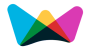#Distance Between 2 Points Calculator

This tool will helps you to find the distance between two given points on a line.

Step by Step calculation

## Distance Between Two Points Formula

For any 2 points there is exactly one line segment connecting them. The distance between 2 points is the length of the line segment connecting them.

Distance =  (x2 - x1)2 + (y2 - y1)2

Where

x1,x2 = x Axis Point

y1,y2 = y Axis Point

###See Also ►

Distance Between Two MidPoints

Short Distance Between Two Lines

Distance Between Two Points

Other Calculators

###More Calculators ►

Ordering Fraction Calculator

Find Missing Fraction Calculator

Simplify Fraction Calculator

Fraction Comparison Calculator